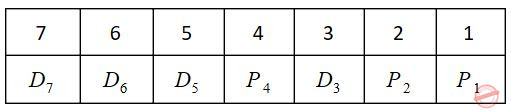October 11, 2023
October 11, 2023
October 11, 2023
###### UGC NET CS 2010 Dec-Paper-2
October 11, 2023
 Question 601
Encoding of data bits 0011 into 7-bit even Parity Hamming Code is
 A 0011110 B 0101110 C 0010110 D 0011100
Question 601 Explanation:
(m) Data = 0011
No. of parity bits needed is decided using
2p≥ m+p+1
p=3
Bit pattern:P1(check even parity at 1, 3, 5, 7 bit) = 0
P2(check even parity at 2, 3, 6, 7 bit) = 1
P4(check even parity at 4, 5, 6, 7 bit) = 1
So encoded data = 0011110

Question 601 Explanation:
(m) Data = 0011
No. of parity bits needed is decided using
2p≥ m+p+1
p=3
Bit pattern:P1(check even parity at 1, 3, 5, 7 bit) = 0
P2(check even parity at 2, 3, 6, 7 bit) = 1
P4(check even parity at 4, 5, 6, 7 bit) = 1
So encoded data = 0011110